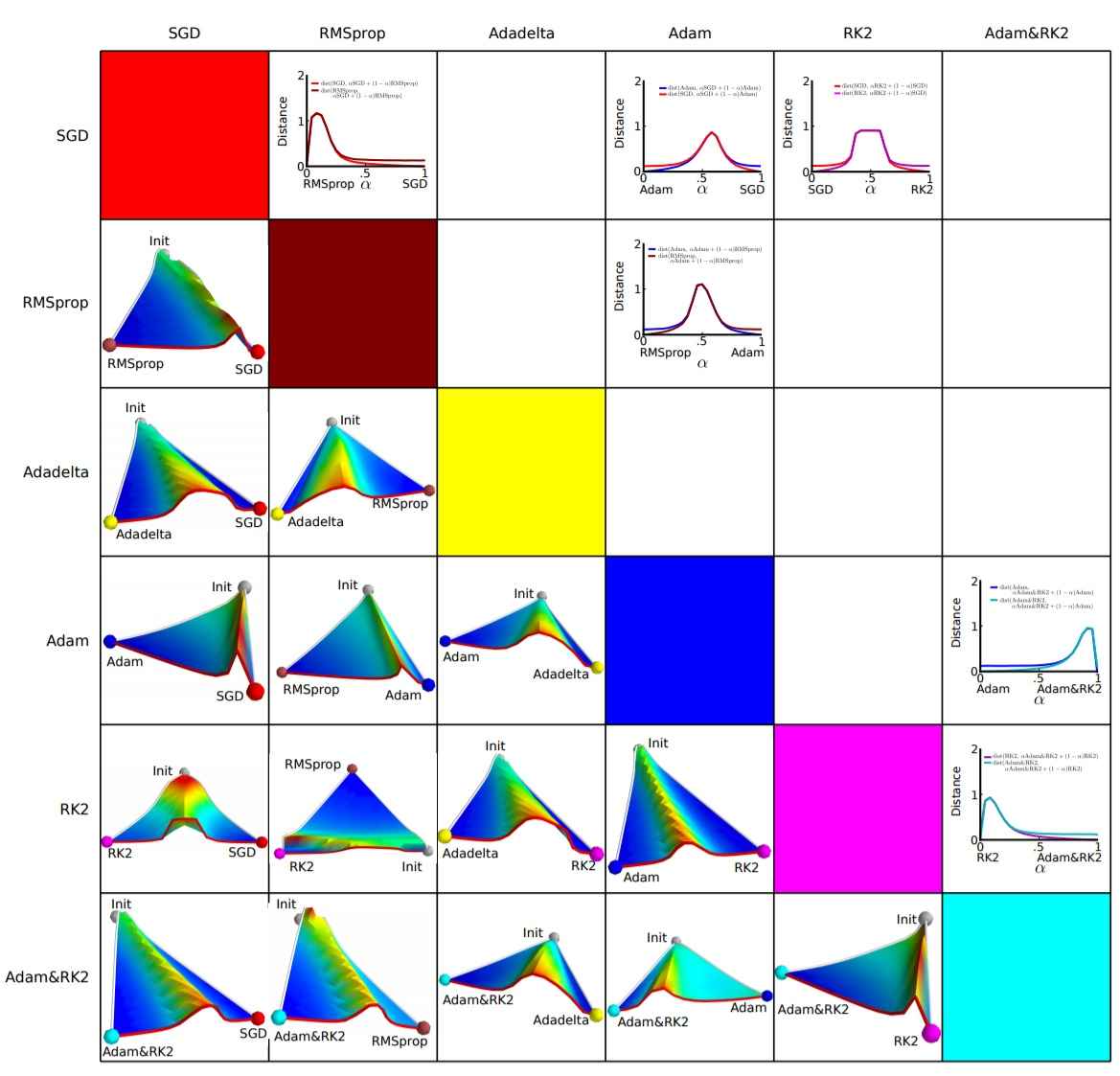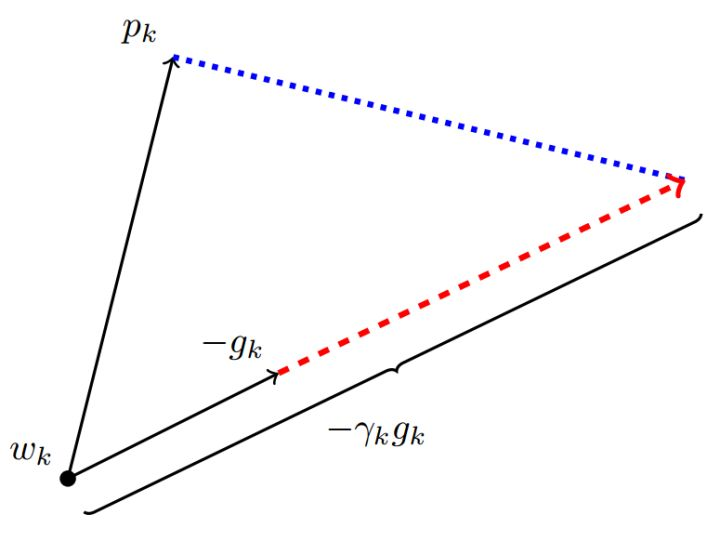Despite the fact that our experimental evidence demonstrates that adaptive methods are not advantageous for machine learning, the Adam algorithm remains incredibly popular. We are not sure exactly as to why ……

## 一个框架回顾优化算法

1. 计算目标函数关于当前参数的梯度： $g_t=\nabla f(w_t)$
2. 根据历史梯度计算一阶动量和二阶动量：$m_t = \phi(g_1, g_2, \cdots, g_t)$; $V_t = \psi(g_1, g_2, \cdots, g_t)$
3. 计算当前时刻的下降梯度： $\eta_t = \alpha \cdot m_t / \sqrt{V_t}$
4. 根据下降梯度进行更新： $w_{t+1} = w_t - \eta_t$

### SGD

$m_t = g_t$
$V_t = I^2$

$\eta_t = \alpha \cdot g_t$

SGD最大的缺点是下降速度慢，而且可能会在沟壑的两边持续震荡，停留在一个局部最优点。

### SGD with Momentum

$m_t = \beta_1 \cdot m_{t-1} + (1-\beta_1)\cdot g_t$

### SGD with Nesterov Acceleration

SGD 还有一个问题是困在局部最优的沟壑里面震荡。想象一下你走到一个盆地，四周都是略高的小山，你觉得没有下坡的方向，那就只能待在这里了。可是如果你爬上高地，就会发现外面的世界还很广阔。因此，我们不能停留在当前位置去观察未来的方向，而要向前一步、多看一步、看远一些。

NAG 全称 Nesterov Accelerated Gradient，是在 SGD、SGD-M 的基础上的进一步改进，改进点在于步骤1。我们知道在时刻 t 的主要下降方向是由累积动量决定的，自己的梯度方向说了也不算，那与其看当前梯度方向，不如先看看如果跟着累积动量走了一步，那个时候再怎么走。因此，NAG在步骤 1，不计算当前位置的梯度方向，而是计算如果按照累积动量走了一步，那个时候的下降方向

$g_t=\nabla f(w_t-\alpha \cdot m_{t-1} / \sqrt{V_{t-1}})$

$V_t = \sum_{\tau=1}^{t} g_\tau^2$

$\eta_t = \alpha \cdot m_t / \sqrt{V_t}$

$V_t = \beta_2 * V_{t-1} + (1-\beta_2) g_t^2$

SGD的一阶动量：

$m_t = \beta_1 \cdot m_{t-1} + (1-\beta_1)\cdot g_t$

$V_t = \beta_2 * V_{t-1} + (1-\beta_2) g_t^2$

$g_t=\nabla f(w_t-\alpha \cdot m_{t-1} / \sqrt{V_t})$

$\eta_t = \alpha / \sqrt{V_t}$

$V_t = max(\beta_2 * V_{t-1} + (1-\beta_2) g_t^2, V_{t-1})$

## 优化算法的选择与使用策略

### 不同优化算法的核心差异

$\eta_t = (\alpha/ \sqrt{V_t} ) \cdot m_t$$\eta_t^{Adam} = (\alpha/ \sqrt{V_t} ) \cdot m_t$

$\eta_t^{SGD} = \alpha^{SGD} \cdot g_t$

$\eta_t^{SGD}$ 必定可以分解为 $\eta_t^{Adam}$ 所在方向及其正交方向上的两个方向之和，如下图所示，这里p为Adam下降方向，g为梯度方向，r为SGD的学习率。$proj_{\eta_t^{SGD}} = \eta_t^{Adam}$

$\alpha_t^{SGD} = ((\eta_t^{Adam})^T\eta_t^{Adam})/((\eta_t^{Adam})^Tg_t)$

$\lambda_t^{SGD} = \beta_2 \cdot \lambda_{t-1}^{SGD}+(1-\beta_2) \cdot \alpha_t^{SGD}$

$\tilde{\lambda}_t^{SGD}=\lambda_t^{SGD}/(1-\beta_2^t)$

$|\tilde{\lambda}_t^{SGD} - \alpha_t^{SGD}|<\epsilon$. 每次迭代玩都计算一下SGD接班人的相应学习率，如果发现基本稳定了，那就 SGD 以 $\tilde{\lambda}_t^{SGD}$ 为学习率接班前进。

### 优化算法的常用tricks

1. 首先，各大算法孰优孰劣并无定论。如果是刚入门，优先考虑 SGD+Nesterov Momentum 或者 Adam.（Standford 231n : The two recommended updates to use are either SGD+Nesterov Momentum or Adam）

2. 选择你熟悉的算法——这样你可以更加熟练地利用你的经验进行调参

3. 充分了解你的数据——如果数据是非常稀疏的，那么优先考虑自适应学习率的算法。